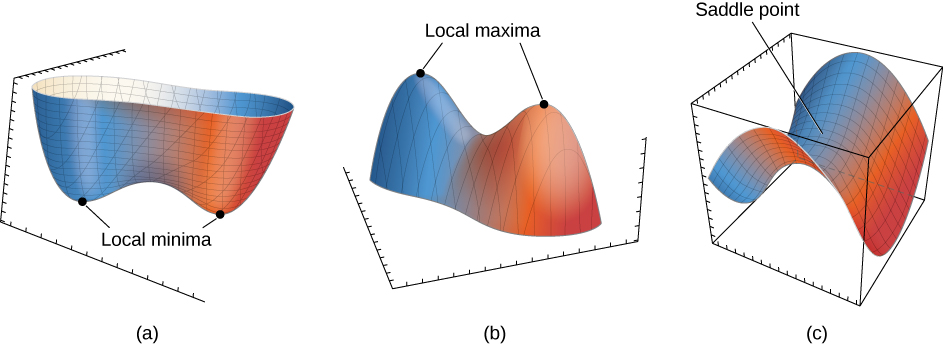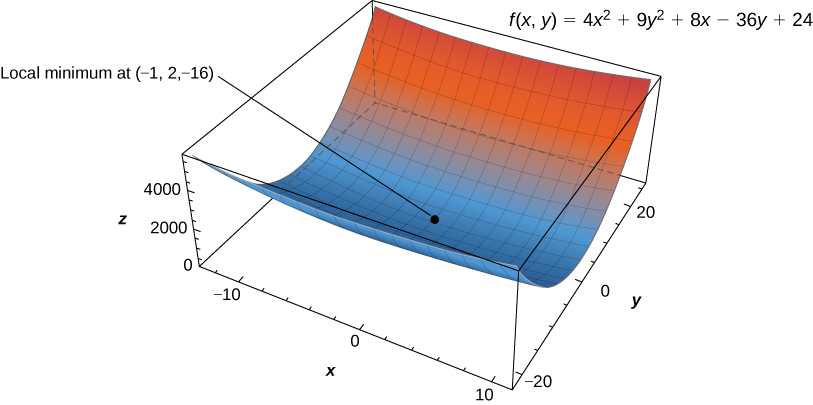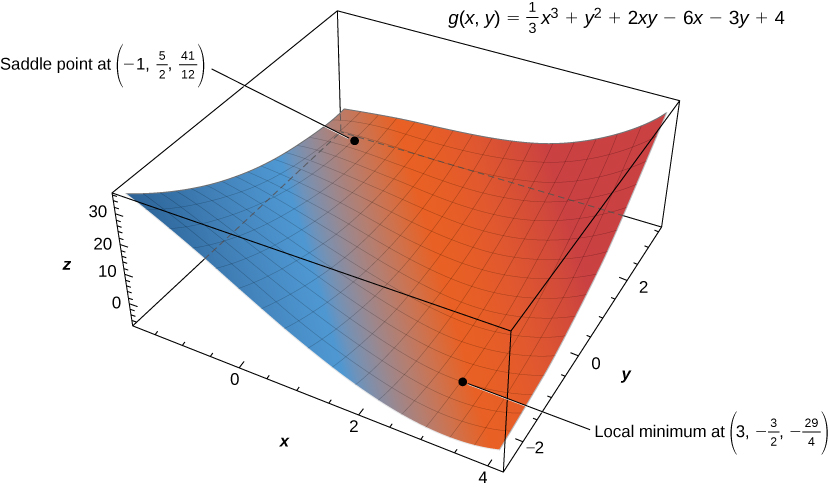# 4.7 Maxima/minima problems  (Page 3/10)

 Page 3 / 10

The second derivative test for a function of one variable provides a method for determining whether an extremum occurs at a critical point of a function. When extending this result to a function of two variables, an issue arises related to the fact that there are, in fact, four different second-order partial derivatives, although equality of mixed partials reduces this to three. The second derivative test for a function of two variables, stated in the following theorem, uses a discriminant     $D$ that replaces $f\text{″}\left({x}_{0}\right)$ in the second derivative test for a function of one variable.

## Second derivative test

Let $z=f\left(x,y\right)$ be a function of two variables for which the first- and second-order partial derivatives are continuous on some disk containing the point $\left({x}_{0},{y}_{0}\right).$ Suppose ${f}_{x}\left({x}_{0},{y}_{0}\right)=0$ and ${f}_{y}\left({x}_{0},{y}_{0}\right)=0.$ Define the quantity

$D={f}_{xx}\left({x}_{0},{y}_{0}\right){f}_{yy}\left({x}_{0},{y}_{0}\right)-{\left({f}_{xy}\left({x}_{0},{y}_{0}\right)\right)}^{2}.$
1. If $D>0$ and ${f}_{xx}\left({x}_{0},{y}_{0}\right)>0,$ then $f$ has a local minimum at $\left({x}_{0},{y}_{0}\right).$
2. If $D>0$ and ${f}_{xx}\left({x}_{0},{y}_{0}\right)<0,$ then $f$ has a local maximum at $\left({x}_{0},{y}_{0}\right).$
3. If $D<0,,$ then $f$ has a saddle point at $\left({x}_{0},{y}_{0}\right).$
4. If $D=0,$ then the test is inconclusive.The second derivative test can often determine whether a function of two variables has a local minima (a), a local maxima (b), or a saddle point (c).

To apply the second derivative test, it is necessary that we first find the critical points of the function. There are several steps involved in the entire procedure, which are outlined in a problem-solving strategy.

## Problem-solving strategy: using the second derivative test for functions of two variables

Let $z=f\left(x,y\right)$ be a function of two variables for which the first- and second-order partial derivatives are continuous on some disk containing the point $\left({x}_{0},{y}_{0}\right).$ To apply the second derivative test to find local extrema, use the following steps:

1. Determine the critical points $\left({x}_{0},{y}_{0}\right)$ of the function $f$ where ${f}_{x}\left({x}_{0},{y}_{0}\right)={f}_{y}\left({x}_{0},{y}_{0}\right)=0.$ Discard any points where at least one of the partial derivatives does not exist.
2. Calculate the discriminant $D={f}_{xx}\left({x}_{0},{y}_{0}\right){f}_{yy}\left({x}_{0},{y}_{0}\right)-{\left({f}_{xy}\left({x}_{0},{y}_{0}\right)\right)}^{2}$ for each critical point of $f.$
3. Apply [link] to determine whether each critical point is a local maximum, local minimum, or saddle point, or whether the theorem is inconclusive.

## Using the second derivative test

Find the critical points for each of the following functions, and use the second derivative test to find the local extrema:

1. $f\left(x,y\right)=4{x}^{2}+9{y}^{2}+8x-36y+24$
2. $g\left(x,y\right)=\frac{1}{3}{x}^{3}+{y}^{2}+2xy-6x-3y+4$
1. Step $1$ of the problem-solving strategy involves finding the critical points of $f.$ To do this, we first calculate ${f}_{x}\left(x,y\right)$ and ${f}_{y}\left(x,y\right),$ then set each of them equal to zero:
$\begin{array}{ccc}\hfill {f}_{x}\left(x,y\right)& =\hfill & 8x+8\hfill \\ \hfill {f}_{y}\left(x,y\right)& =\hfill & 18y-36.\hfill \end{array}$

Setting them equal to zero yields the system of equations
$\begin{array}{ccc}\hfill 8x+8& =\hfill & 0\hfill \\ \hfill 18y-36& =\hfill & 0.\hfill \end{array}$

The solution to this system is $x=-1$ and $y=2.$ Therefore $\left(-1,2\right)$ is a critical point of $f.$
Step 2 of the problem-solving strategy involves calculating $D.$ To do this, we first calculate the second partial derivatives of $f\text{:}$
$\begin{array}{ccc}\hfill {f}_{xx}\left(x,y\right)& =\hfill & 8\hfill \\ \hfill {f}_{xy}\left(x,y\right)& =\hfill & 0\hfill \\ \hfill {f}_{yy}\left(x,y\right)& =\hfill & 18.\hfill \end{array}$

Therefore, $D={f}_{xx}\left(-1,2\right){f}_{yy}\left(-1,2\right)-{\left({f}_{xy}\left(-1,2\right)\right)}^{2}=\left(8\right)\left(18\right)-{\left(0\right)}^{2}=144.$
Step 3 states to check [link] . Since $D>0$ and ${f}_{xx}\left(-1,2\right)>0,$ this corresponds to case 1. Therefore, $f$ has a local minimum at $\left(-1,2\right)$ as shown in the following figure.The function f ( x , y ) has a local minimum at ( −1 , 2 , −16 ) .
2. For step 1, we first calculate ${g}_{x}\left(x,y\right)$ and ${g}_{y}\left(x,y\right),$ then set each of them equal to zero:
$\begin{array}{rrr}{g}_{x}\left(x,y\right)& =\hfill & {x}^{2}+2y-6\hfill \\ \hfill {g}_{y}\left(x,y\right)& =\hfill & 2y+2x-3.\hfill \end{array}$

Setting them equal to zero yields the system of equations
$\begin{array}{ccc}\hfill {x}^{2}+2y-6& =\hfill & 0\hfill \\ \hfill 2y+2x-3& =\hfill & 0.\hfill \end{array}$

To solve this system, first solve the second equation for y. This gives $y=\frac{3-2x}{2}.$ Substituting this into the first equation gives
$\begin{array}{ccc}\hfill {x}^{2}+3-2x-6& =\hfill & 0\hfill \\ \hfill {x}^{2}-2x-3& =\hfill & 0\hfill \\ \hfill \left(x-3\right)\left(x+1\right)& =\hfill & 0.\hfill \end{array}$

Therefore, $x=-1$ or $x=3.$ Substituting these values into the equation $y=\frac{3-2x}{2}$ yields the critical points $\left(-1,\frac{5}{2}\right)$ and $\left(3,-\frac{3}{2}\right).$

Step 2 involves calculating the second partial derivatives of $g\text{:}$

$\begin{array}{ccc}\hfill {g}_{xx}\left(x,y\right)& =\hfill & 2x\hfill \\ \hfill {g}_{xy}\left(x,y\right)& =\hfill & 2\hfill \\ \hfill {g}_{yy}\left(x,y\right)& =\hfill & 2.\hfill \end{array}$

Then, we find a general formula for $D\text{:}$
$\begin{array}{cc}\hfill D& ={g}_{xx}\left({x}_{0},{y}_{0}\right){g}_{yy}\left({x}_{0},{y}_{0}\right)-{\left({g}_{xy}\left({x}_{0},{y}_{0}\right)\right)}^{2}\hfill \\ & =\left(2{x}_{0}\right)\left(2\right)-{2}^{2}\hfill \\ & =4{x}_{0}-4.\hfill \end{array}$

Next, we substitute each critical point into this formula:
$\begin{array}{ccc}\hfill D\left(-1,\frac{5}{2}\right)& =\hfill & \left(2\left(-1\right)\right)\left(2\right)-{\left(2\right)}^{2}=-4-4=-8\hfill \\ \hfill D\left(3,-\frac{3}{2}\right)& =\hfill & \left(2\left(3\right)\right)\left(2\right)-{\left(2\right)}^{2}=12-4=8.\hfill \end{array}$

In step 3, we note that, applying [link] to point $\left(-1,\frac{5}{2}\right)$ leads to case $3,$ which means that $\left(-1,\frac{5}{2}\right)$ is a saddle point. Applying the theorem to point $\left(3,-\frac{3}{2}\right)$ leads to case 1, which means that $\left(3,-\frac{3}{2}\right)$ corresponds to a local minimum as shown in the following figure.The function g ( x , y ) has a local minimum and a saddle point.

are nano particles real
yeah
Joseph
Hello, if I study Physics teacher in bachelor, can I study Nanotechnology in master?
no can't
Lohitha
where we get a research paper on Nano chemistry....?
nanopartical of organic/inorganic / physical chemistry , pdf / thesis / review
Ali
what are the products of Nano chemistry?
There are lots of products of nano chemistry... Like nano coatings.....carbon fiber.. And lots of others..
learn
Even nanotechnology is pretty much all about chemistry... Its the chemistry on quantum or atomic level
learn
da
no nanotechnology is also a part of physics and maths it requires angle formulas and some pressure regarding concepts
Bhagvanji
hey
Giriraj
Preparation and Applications of Nanomaterial for Drug Delivery
revolt
da
Application of nanotechnology in medicine
has a lot of application modern world
Kamaluddeen
yes
narayan
what is variations in raman spectra for nanomaterials
ya I also want to know the raman spectra
Bhagvanji
I only see partial conversation and what's the question here!
what about nanotechnology for water purification
please someone correct me if I'm wrong but I think one can use nanoparticles, specially silver nanoparticles for water treatment.
Damian
yes that's correct
Professor
I think
Professor
Nasa has use it in the 60's, copper as water purification in the moon travel.
Alexandre
nanocopper obvius
Alexandre
what is the stm
is there industrial application of fullrenes. What is the method to prepare fullrene on large scale.?
Rafiq
industrial application...? mmm I think on the medical side as drug carrier, but you should go deeper on your research, I may be wrong
Damian
How we are making nano material?
what is a peer
What is meant by 'nano scale'?
What is STMs full form?
LITNING
scanning tunneling microscope
Sahil
how nano science is used for hydrophobicity
Santosh
Do u think that Graphene and Fullrene fiber can be used to make Air Plane body structure the lightest and strongest. Rafiq
Rafiq
what is differents between GO and RGO?
Mahi
what is simplest way to understand the applications of nano robots used to detect the cancer affected cell of human body.? How this robot is carried to required site of body cell.? what will be the carrier material and how can be detected that correct delivery of drug is done Rafiq
Rafiq
if virus is killing to make ARTIFICIAL DNA OF GRAPHENE FOR KILLED THE VIRUS .THIS IS OUR ASSUMPTION
Anam
analytical skills graphene is prepared to kill any type viruses .
Anam
Any one who tell me about Preparation and application of Nanomaterial for drug Delivery
Hafiz
what is Nano technology ?
write examples of Nano molecule?
Bob
The nanotechnology is as new science, to scale nanometric
brayan
nanotechnology is the study, desing, synthesis, manipulation and application of materials and functional systems through control of matter at nanoscale
Damian
Is there any normative that regulates the use of silver nanoparticles?
what king of growth are you checking .?
Renato
how did you get the value of 2000N.What calculations are needed to arrive at it
Privacy Information Security Software Version 1.1a
Good
can you provide the details of the parametric equations for the lines that defince doubly-ruled surfeces (huperbolids of one sheet and hyperbolic paraboloid). Can you explain each of the variables in the equations?

#### Get Jobilize Job Search Mobile App in your pocket Now!By Sarah WarrenBy OpenStaxBy David BourgeoisBy Brooke DelaneyBy Edgar DelgadoBy OpenStaxBy Wey HeyBy Brooke DelaneyBy Courntey HubBy Sandhills MLT